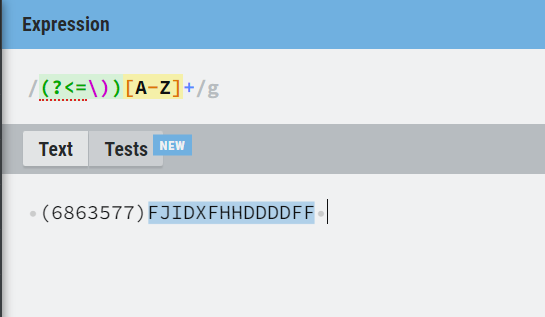# Split the string data

Hai

Here is the string variable " (6863577)FJIDXFHHDDDDFF ". This is the input, i need “FJIDXFHHDDDDFF” As the output.

@Aarthy1

Use regex

System.Text.RegularExpressions.Regex.Match(Input,“(?<=))[A-Z]+”).Value

Hi @Aarthy1

``````- Assign -> StrVar = "(6863577)FJIDXFHHDDDDFF"
- Assign -> StrVar = StrVar.Split(")")(1).toString.trim
``````

Check the below image for better understanding

You can use the below regular expression to extract the output.

``````System.Text.RegularExpressions.Regex.Matches(yourstringinput.ToString,“((?<=\))[A-Z]+)”)
``````Hope it helps!!

1 Like

Hi @Aarthy1

``````string input = "(6863577)FJIDXFHHDDDDFF";
string output = input.Substring(input.IndexOf(")") + 1)

``````

Hope it helps!!

1 Like4 Likes

Hey @Aarthy1 ,
You can use` input.Split(")"c)(1)`

So basically the above code will split the string on the basis of closing parenthesis , i.e. “)”

The output of normal split is a collection, but we want a string after the closing parenthesis so we write (1) to access the second element (index 1) of the array that results from the split. Arrays

below is the output

Hope it helps you

2 Likes

If your input string is the same format as (6863577)FJIDXFHHDDDDFF , you can [A-Z]+ regex expression

outputString = regex.match(inputString,“[A-Z]+”)